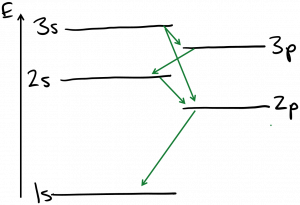Laser Photonics Optical transitions in atoms

# Optical transitions in atoms

As we’ve seen, electron states in atoms occur at discrete energies, and each state is described by a unique set of four quantum numbers:$n, l, m$ and$s$.  Due to the fact that an electron is a Fermion, only one electron can be found in each state within an atom at any given time; this is Pauli’s exclusion principle.  In equilibrium, electrons fill the energy states in an atom starting from the lowest energy state$n = 1$ and working upwards in energy.

If light was purely a wave, the energy it carries would be continuously variable with the amplitude (intensity) of the light.  But experiments such as the photoelectric effect establish that this is not the case.  In the photoelectric experiment, light shining on a metal surface delivers energy to electrons in the metal.  An electron that has absorbed sufficient energy to overcome the electrostatic attraction to the metal nuclei will be ejected from the metal surface.  If light was purely a wave, the rate of electrons ejected would depend solely on the intensity of the light.  However, it is observed that no electrons are ejected unless the frequency of the light is above a certain threshold.  From this, it is deduced that electrons (usually) absorb a single chunk of energy, called a photon, from the light wave; and the photon has energy related to the frequency of the light:$\displaystyle E = h \nu$

Let’s go back to our atom.  If an electron sitting in a particular energy state$E_{start}$ in the atom absorbs a photon, the photon is entirely destroyed, and conservation of energy tells us that the electron must gain energy equal to that of the photon.  However, the electron can only do this if there exists another energy state such that$E_{final} = E_{start} + h \nu$.  Therefore, the electron can only absorb photons whose frequency corresponds to the energy difference between electron energy states.  This is also true for photon emission, where an electron drops to a lower energy state and creates a photon whose energy equals the energy lost by the electron.  Thus, the visible spectrum of light emitted by hydrogen atoms consists of four ‘lines’ at discrete wavelengths: 410 nm, 434 nm, 486 nm, and 656 nm, as shown at the following link:  https://en.wikipedia.org/wiki/Balmer_series

As it transpires, there are additional restrictions on these photon absorption and emission events.  Not all transitions between energy states are physically likely to occur, because energy isn’t the only quantity that must be conserved.  Photons are spin-1 particles, and conservation of angular momentum tells us that the total angular momentum of the starting and ending electron states must differ by 1.  This is most commonly satisfied by making transitions between states whose orbital angular momentum quantum number$l$ differs by 1.  These types of rules governing allowed and disallowed transitions are called selection rules.  Below is a sketch of the energy levels of neon atoms (used in He-Ne lasers) with the allowed optical transitions labeled.